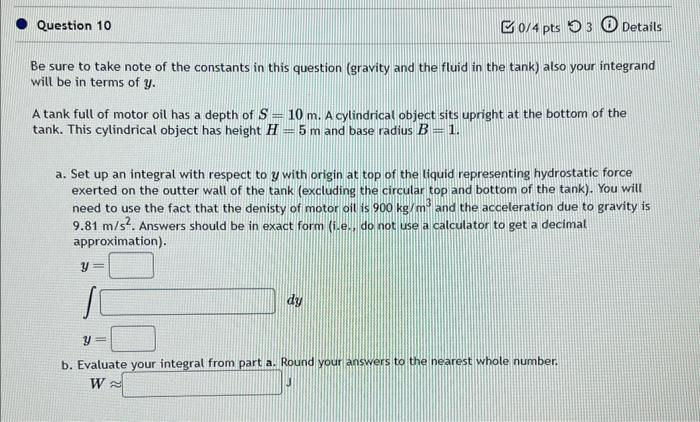# Question be sure to take note of the constants in this question (gravity and the fluid in the tank) also your integrand vill be in terms of $$y$$. A tank full of motor oil has a depth of $$S=10 \mathrm{~m}$$. A cylindrical object sits upright at the bottom of the tank. This cylindrical object has height $$H=5 \mathrm{~m}$$ and base radius $$B=1$$. a. Set up an integral with respect to $$y$$ with origin at top of the liquid representing hydrostatic force exerted on the outter wall of the tank (excluding the circular top and bottom of the tank). You will need to use the fact that the denisty of motor oit is $$900 \mathrm{~kg} / \mathrm{m}^{3}$$ and the acceleration due to gravity is $$9.81 \mathrm{~m} / \mathrm{s}^{2}$$. Answers should be in exact form (i.e.. do not use a calculator to get a decimal approximation). $\begin{array}{l} y= \\ y= \\ y= \end{array} d y$ b. Evaluate vour integral from part a. Round your answers to the nearest whole number. $W \approx$Transcribed Image Text: be sure to take note of the constants in this question (gravity and the fluid in the tank) also your integrand vill be in terms of $$y$$. A tank full of motor oil has a depth of $$S=10 \mathrm{~m}$$. A cylindrical object sits upright at the bottom of the tank. This cylindrical object has height $$H=5 \mathrm{~m}$$ and base radius $$B=1$$. a. Set up an integral with respect to $$y$$ with origin at top of the liquid representing hydrostatic force exerted on the outter wall of the tank (excluding the circular top and bottom of the tank). You will need to use the fact that the denisty of motor oit is $$900 \mathrm{~kg} / \mathrm{m}^{3}$$ and the acceleration due to gravity is $$9.81 \mathrm{~m} / \mathrm{s}^{2}$$. Answers should be in exact form (i.e.. do not use a calculator to get a decimal approximation). $\begin{array}{l} y= \\ y= \\ y= \end{array} d y$ b. Evaluate vour integral from part a. Round your answers to the nearest whole number. $W \approx$
Transcribed Image Text: be sure to take note of the constants in this question (gravity and the fluid in the tank) also your integrand vill be in terms of $$y$$. A tank full of motor oil has a depth of $$S=10 \mathrm{~m}$$. A cylindrical object sits upright at the bottom of the tank. This cylindrical object has height $$H=5 \mathrm{~m}$$ and base radius $$B=1$$. a. Set up an integral with respect to $$y$$ with origin at top of the liquid representing hydrostatic force exerted on the outter wall of the tank (excluding the circular top and bottom of the tank). You will need to use the fact that the denisty of motor oit is $$900 \mathrm{~kg} / \mathrm{m}^{3}$$ and the acceleration due to gravity is $$9.81 \mathrm{~m} / \mathrm{s}^{2}$$. Answers should be in exact form (i.e.. do not use a calculator to get a decimal approximation). $\begin{array}{l} y= \\ y= \\ y= \end{array} d y$ b. Evaluate vour integral from part a. Round your answers to the nearest whole number. $W \approx$&#12304;General guidance&#12305;The answer provided below has been developed in a clear step by step manner.Step1/2(a) To set up an integral with respect to y representing hydrostatic force exerted on the outer wall of the tank, we need to consider an infinitesimal horizontal strip of the tank at a depth y from the top. The width of this strip is the circumference of the cylindrical object, which is 2&#960;B. The thickness of the strip is dy. The hydrostatic force on this strip is the product of the pressure and the area, which is given by:$$\mathrm{{d}{F}=ρ{g}{h}\times{2}π{B}{\left.{d}{y}\right.}}$$where &#961; is the density of the motor oil, g is the acceleration due to gravity, and h is the depth of the strip from the top of the liquid. The total hydrostatic force on the outer wall of the tank is obtained by integrating this expression from y = 0 to y = S:\( \mathrm{{F}=∫{\left({0}\to{S}\right)}{d}{F}=∫{\left ... See the full answer